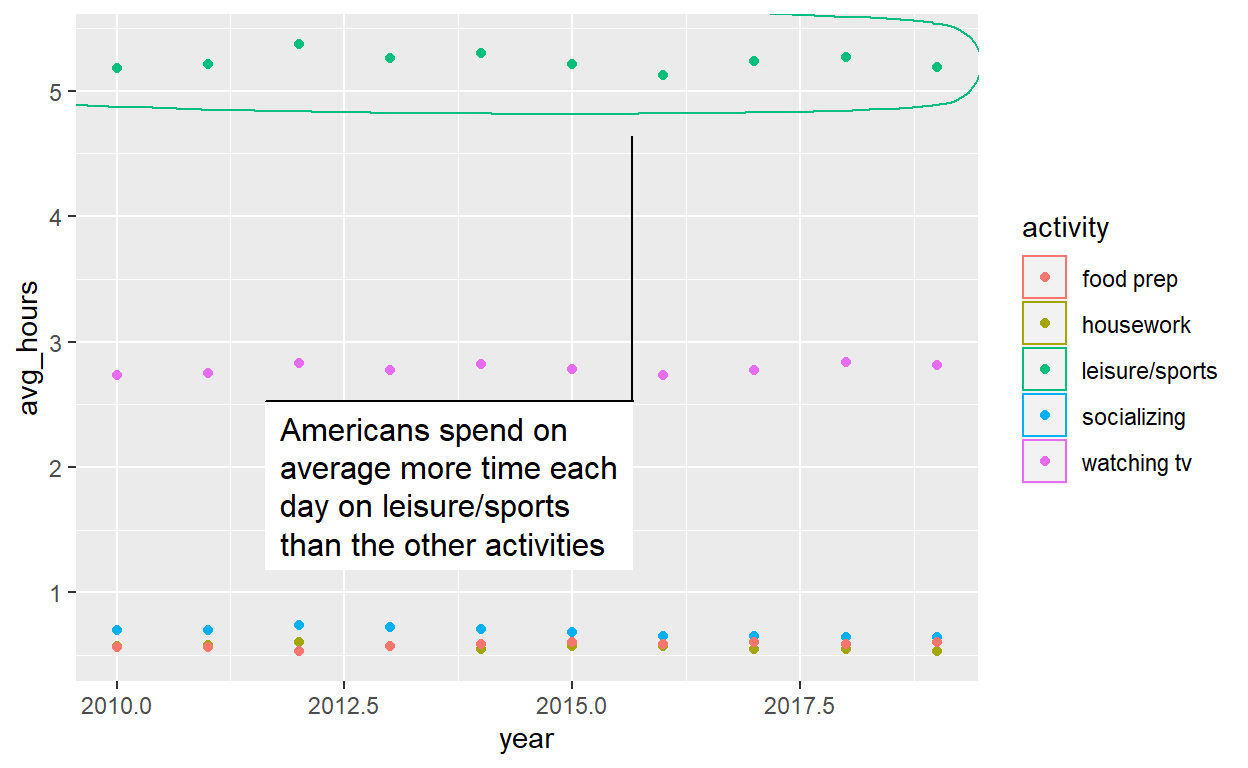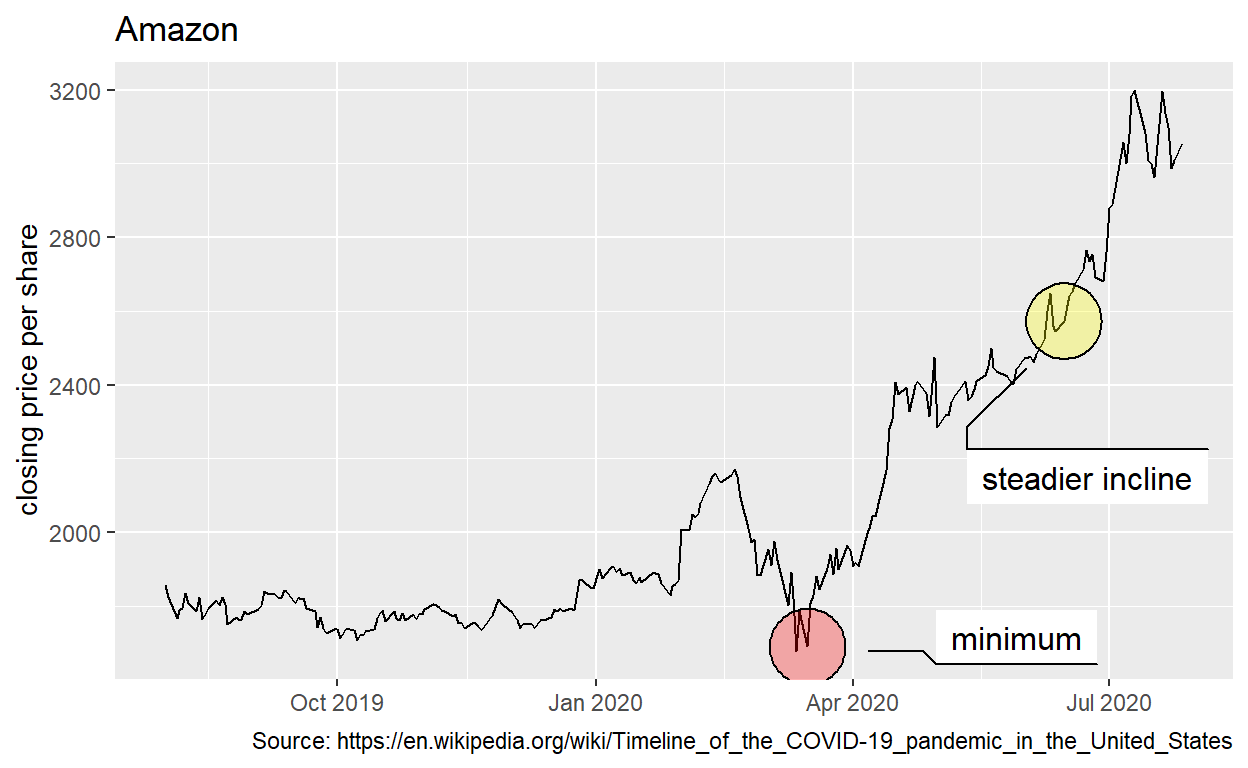# Data Visualization

Code for quiz 9

1. Load the packages we will use.
``````library(tidyverse)
library(echarts4r)
library(ggforce)
library(tidyquant)
``````

# Question: e_charts-1

Create a bar chart that shows the average hours Americans spend on five activities by year. Use the timeline argument to create an animation that will animate through the years,

• spend_time contains 10 years of data on how many hours americans spend each day on 5 activities

``````spend_time  <- read.csv("https://estanny.com/static/week8/spend_time.csv")
``````

e_charts-1

Start with `spend_time`

• THEN group_by `year`
• THEN create an e_chart that assigns `activity` to the x-axis and will show activity by `year`
• THEN use `e_timeline_opts` to set autoPlay to TRUE
• THEN use `e_bar` to represent the variable `avg_hours` with a bar chart
• THEN use `e_title` to set the main title to ‘Average hours Americans spend per day on each activity’
• THEN remove the legend with `e_legend`
``````spend_time  %>%
group_by(year)  %>%
e_charts(x = activity, timeline = TRUE)  %>%
e_timeline_opts(autoPlay = TRUE)  %>%
e_bar(serie = avg_hours)  %>%
e_title(text = 'Average hours Americans spend per day on each activity')  %>%
e_legend(show = FALSE)
``````

# Question: echarts-2

Create a line chart for the activities that Americans spend time on.

Start with `spend_time`

• THEN use `mutate` to convert `year` from a number to a string (year-month-day) using `mutate`
• first convert `year` to a string “201X-12-31” using the function `paste`
• `paste` will paste each year to 12 and 31 THEN
• THEN use `mutate` to convert year from a character object to a date object using the `ymd` function from the `lubridate` package. `ymd` converts dates stored as characters to date objects.
• THEN `group_by` the variable `activity`
• THEN initiate an `e_charts` object with `year` on the x-axis
• THEN use `e_line` to add a line to the variable `avg_hours`
• THEN add a tooltip with `e_tooltip`
• THEN use `e_title` to set the main title to ‘Average hours Americans spend per day on each activity’
• THEN use `e_legend(top = 40)` to move the legend down

# Question 3: Modify slide 82

• Create a plot with `spend_time` data
• assign `year` to the x-axis
• assign `avg_hours` to the y-axis
• assign `activity` to color
• ADD points with `geom_point`
• ADD `geom_mark_ellipse`
• filter on activity == “leisure/sports”
• description is “Americans spend the most time on leisure/sport”
``````ggplot(spend_time, aes(x = year, y = avg_hours, color = activity)) +
geom_point() +
geom_mark_ellipse(aes(filter = activity == "leisure/sports", description = "Americans spend on average more time each day on leisure/sports than the other activities"))
``````# Question: tidyquant

``````library(tidyquant)
``````

Retrieve stock price for Amazon, ticker AMZN using `tq_get`

• from 2019-08-01 to 2020-07-28
• assign output to `df`
``````df  <- tq_get("AMZN", get = "stock.prices", from = "2019-08-01", to = "2020-07-28" )
``````

create a plot with the `df`data

• assign `date` to the x-axis
• assign `close` to the y-axis
• ADD a line with `geom_line`
• ADD `geom_mark_ellipse`
• filter on a date to mark. Pick a date after looking at the line plot. Include the date in your Rmd code chunk.
• include a description of something that happened on that date from the pandemic timeline. Include the description in your Rmd code chunk
• fill the ellipse yellow
• ADD `geom_mark_ellipse`
• filter on the date that had the minimum `close` price. Include the date in your Rmd code chunk.
• include a description of something that happened on that date from the pandemic timeline. Include the description in your Rmd code chunk
• color the ellipse red
• ADD `labs`
``````ggplot(df, aes(x = date, y = close)) +
geom_line() +
geom_mark_ellipse(aes(filter = date == "2020-06-15", description = "steadier incline"), fill = "yellow") +
geom_mark_ellipse(aes(filter = date == "2020-03-16", description = "minimum"), fill = "red") +
labs(title = "Amazon",
x = NULL,
y = "closing price per share",
caption = "Source: https://en.wikipedia.org/wiki/Timeline_of_the_COVID-19_pandemic_in_the_United_States")
````````````ggsave(filename= "preview.png",
path = here::here("_posts", "2021-04-20-data-visualization"))
``````